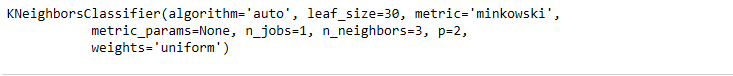# Saving a machine learning Model

• Difficulty Level : Easy
• Last Updated : 24 Jun, 2021

In machine learning, while working with scikit learn library, we need to save the trained models in a file and restore them in order to reuse it to compare the model with other models, to test the model on a new data. The saving of data is called Serialization, while restoring the data is called Deserialization.
Also, we deal with different types and sizes of data. Some datasets are easily trained i.e- they take less time to train but the datasets whose size is large (more than 1GB) can take very large time to train on a local machine even with GPU. When we need the same trained data in some different project or later sometime, to avoid the wastage of the training time, store trained model so that it can be used anytime in the future.
There are two ways we can save a model in scikit learn:

1. Pickle string: The pickle module implements a fundamental, but powerful algorithm for serializing and de-serializing a Python object structure.

Pickle model provides the following functions –
pickle.dump to serialize an object hierarchy, you simply use dump().

Attention reader! Don’t stop learning now. Get hold of all the important Machine Learning Concepts with the Machine Learning Foundation Course at a student-friendly price and become industry ready.

1. Example: Let’s apply K Nearest Neighbor on iris dataset and then save the model.

## Python3

 `import` `numpy as np` `# Load dataset``from` `sklearn.datasets ``import` `load_iris``iris ``=` `load_iris()` `X ``=` `iris.data``y ``=` `iris.target` `# Split dataset into train and test``X_train, X_test, y_train, y_test ``=` `\``    ``train_test_split(X, y, test_size ``=` `0.3``,``                        ``random_state ``=` `2018``)` `# import KNeighborsClassifier model``from` `sklearn.neighbors ``import` `KNeighborsClassifier as KNN``knn ``=` `KNN(n_neighbors ``=` `3``)` `# train model``knn.fit(X_train, y_train)`
1.1. Save model to string using pickle –

## Python3

 `import` `pickle` `# Save the trained model as a pickle string.``saved_model ``=` `pickle.dumps(knn)` `# Load the pickled model``knn_from_pickle ``=` `pickle.loads(saved_model)` `# Use the loaded pickled model to make predictions``knn_from_pickle.predict(X_test)`
1. Output:1.
2. Pickled model as a file using joblib: Joblib is the replacement of pickle as it is more efficient on objects that carry large numpy arrays. These functions also accept file-like object instead of filenames.

joblib.dump to serialize an object hierarchy
joblib.load to deserialize a data stream

1. Save to pickled file using joblib –

## Python3

 `from` `sklearn.externals ``import` `joblib` `# Save the model as a pickle in a file``joblib.dump(knn, ``'filename.pkl'``)` `# Load the model from the file``knn_from_joblib ``=` `joblib.load(``'filename.pkl'``)` `# Use the loaded model to make predictions``knn_from_joblib.predict(X_test)`
1. Output:My Personal Notes arrow_drop_up